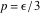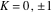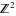# Volume 13

## A Toolbox for Quasirandom Simulation »NBCDFPDF

Published December 30, 2011

Quasirandom simulation uses low-discrepancy or quasirandom sequences in place of pseudorandom sequences, producing faster convergence in problems of moderate dimensions. The objective of this article is both pedagogical and practical: to provide an easily understood introduction to the construction of Sobol sequences and a toolkit for constructing and evaluating such sequences. Read More »

## MathPDE: A Package to Solve PDEs by Finite Differences »NBCDFPDF

Published December 21, 2011

A package for solving time-dependent partial differential equations (PDEs), MathPDE, is presented. It implements finite-difference methods. After making a sequence of symbolic transformations on the PDE and its initial and boundary conditions, MathPDE automatically generates a problem-specific set of Mathematica functions to solve the numerical problem, which is essentially a system of algebraic equations. MathPDE then internally calls MathCode, a Mathematica-to-C++ code generator, to generate a C++ program for solving the algebraic problem, and compiles it into an executable that can be run via MathLink. When the algebraic system is nonlinear, the Newton-Raphson method is used and SuperLU, a library for sparse systems, is used for matrix operations. This article discusses the wide range of PDEs that can be handled by MathPDE, the accuracy of the finite-difference schemes used, and importantly, the ability to handle both regular and irregular spatial domains. Since a standalone C++ program is generated to compute the numerical solution, the package offers portability. Read More »

## Sampling Distribution of ML Estimators: Cauchy Example »NBCDFPDF

Published December 4, 2011

We show how to use the Edgeworth series to construct an accurate approximation to the sampling distribution of the maximum likelihood estimator of a parameter of a Cauchy distribution. We then demonstrate the accuracy of this approximation, valid even for relatively small samples. Read More »

## The Acoustic Wave Equation in the Expanding Universe: Sachs-Wolfe Theorem »NBCDFPDF

Published October 28, 2011

This article considers the acoustic field propagating in the radiation-dominated () universe of arbitrary space curvature (). The field equations are reduced to the d’Alembert equation in an auxiliary static Robertson-Walker spacetime and dispersion relations are discussed. Read More »

## Computing Mixed-Design (Split-Plot) ANOVA »NBCDFPDF

Published October 5, 2011

The mixed, within-between subjects ANOVA (also called a split-plot ANOVA) is a statistical test of means commonly used in the behavioral sciences. One approach to computing this analysis is to use a corrected between-subjects ANOVA. A second approach uses the general linear model by partitioning the sum of squares and cross-product matrices. Both approaches are detailed in this article. Finally, a package called MixedDesignANOVA is introduced that runs mixed-design ANOVAs using the second approach and displays summary statistics as well as a mean plot. Read More »

## Two Basic Results Concerning Random Walks on Graphs »NBCDFPDF

Published September 21, 2011

Two problems involving random walks on graphs are studied. First, the starting point from which a random walk onis most likely to hit a given point before another given point is determined. Second, the slowest mixing initial distribution under a random walk on a given finite graph is found. Read More »

## Betting Two Patterns against Each Other »NBCDFPDF

Published September 1, 2011

We present a technique for computing the probability that a specific pattern of successes and failures is generated randomly before another such pattern, thus winning the corresponding game. The program we build for this purpose finds the mean and standard deviation of the number of trials needed to complete one round of such a game. It can be used to maximize the probability of winning a game by choosing the best possible pattern, and also by adjusting the probability of a success. Finally, we verify our theoretical results by a Monte Carlo simulation. Read More »

## A Study of Super-Nonlinear Motion of a Simple Pendulum »NBCDFPDF

Published August 9, 2011

Two identically charged simple pendulums are allowed to swing in a vertical plane, acted upon by gravity and their mutual electrostatic interactive repulsive forces. We show that the inclusion of the electrostatic interaction makes the movement of the pendulums highly nonlinear. To describe the motion, we quantify the relevant time-dependent kinematic and dynamic quantities. Our analysis also includes an extended study of equal and oppositely charged pendulums. Motivated by the outcomes of the calculation, the author manufactured a real-life replica of the study demonstrating the features of the interactive pendulums; a photograph of the replica is included. Read More »

## Fisher Discrimination with Kernels »NBCDFPDF

Published July 26, 2011

Fisher first introduced the Fisher linear discriminant back in 1938. After the popularization of the support vector machine (SVM) and the kernel trick it became inevitable that the Fisher linear discriminant would be kernelized. Sebastian Mika accomplished this task as part of his Ph.D. in 2002 and the kernelized Fisher discriminant (KFD) now forms part of the large-scale machine-learning tool Shogun. In this article we introduce the package MathKFD. We apply MathKFD to synthetic datasets to demonstrate nonlinear classification via kernels. We also test performance on datasets from the machine-learning literature. The construction of MathKFD follows closely in style the construction of MathSVM by Nilsson and colleagues. We hope these two packages and others of the same ilk will eventually be integrated to form a kernel-based machine-learning environment for Mathematica. Read More »

## Solving Kepler’s Problem »NBCDFPDF

Published July 20, 2011

We demonstrate how the equation for the location of a satellite orbiting a large primary can be solved with the help of quaternions. We also treat the issue of orbit determination, given (1) a location and a velocity of the satellite; (2) two observations of its location; and (3) three or more values of its position. Read More »